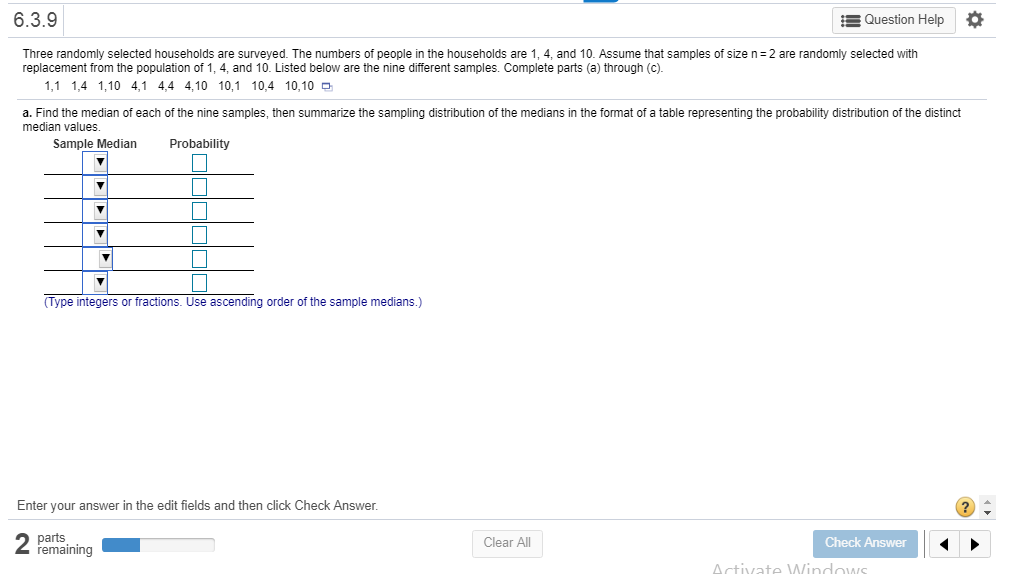# 6.3.9Question HelpThree randomly selected households are surveyed. The numbers of people in the households are 1.4, and 10. Assume that samples of size n = 2 are randomly selected withreplacement from the population of 1, 4, and 10. Listed below are the nine different samples. Complete parts (a) through (c).that1,1 1,4 1,10 4,1 4,4 4,10 10,1 10,4 10,104144 410 101 104 10,10 oe the nine diferent samples. Com le4s anda. Find the median of each of the nine samples, then summarize the sampling distribution of the medians in the format of a table representing the probability distribution of the distinctmedian values.Sample MedianProbabilityType integers or fractions. Use ascending order of the sample medians.)Enter your answer in the edit fields and then click Check AnswerpartsremainingClear AllCheck Answerctivate Windows

Question
21 viewshelp_outlineImage Transcriptionclose6.3.9 Question Help Three randomly selected households are surveyed. The numbers of people in the households are 1.4, and 10. Assume that samples of size n = 2 are randomly selected with replacement from the population of 1, 4, and 10. Listed below are the nine different samples. Complete parts (a) through (c). that 1,1 1,4 1,10 4,1 4,4 4,10 10,1 10,4 10,10 4144 410 101 104 10,10 oe the nine diferent samples. Com le4s and a. Find the median of each of the nine samples, then summarize the sampling distribution of the medians in the format of a table representing the probability distribution of the distinct median values. Sample Median Probability Type integers or fractions. Use ascending order of the sample medians.) Enter your answer in the edit fields and then click Check Answer parts remaining Clear All Check Answer ctivate Windows fullscreen
check_circle

star
star
star
star
star
1 Rating
Step 1

Solution

Step 1:

Given: The nine different random samples of size 2 selected with replacement from a population of 1,4, and 10 are (1,1) (1,4) (1,10) (4, 1) (4, 4) (4, 10) (10, 1) (10, 4) (10,10).

We know the median is the middlemost number in a set of data.  To find the median, we need to arrange the data in the ascending order. Since the sample size is 2 we can find the median of the given samples by taking the average of the two values of each sample. The median for each sample is given in the table below.

Step 2

Step 2:

Now, we need to find the sampling distribution of the median.

To compute the probability of each median value, count the number of particula...

### Want to see the full answer?

See Solution

#### Want to see this answer and more?

Solutions are written by subject experts who are available 24/7. Questions are typically answered within 1 hour.*

See Solution
*Response times may vary by subject and question.
Tagged in

### Measures